# Bihar Board 12th Physics Model Question Paper 4 in English Medium

Bihar Board 12th Physics Model Papers

## Bihar Board 12th Physics Model Question Paper 4 in English Medium

Instructions for the candidates :

1. Candidates are required to give answers in their own words as far as practicable.
2. Figures in the Right-hand margin indicates full marks.
3. While answering the questions, candidates should adhere to the words limit as far as practicable.
4. 15 Minutes of extra time has been allotted to read the questions carefully.
5. This question paper is divided into two sections : Section -A and Section-B
6. In Section A there are 35 Objective type questions which are compulsory, each carrying 1 mark. Darken the circle with Blue/Black ball pen against the correct option on OMR Sheet provided to you. Do not use Whitener/Liquid/ Blade/Nail on OMR Sheet, otherwise, your result will be treated as invalid.
7. In section-B, there are 18 Short answer type questions (each carrying 2 marks), out of which only 10 (ten) questions are to be answered Apart from this there are 06 Long Answer type questions each carrying 5 marks, out of which 3 questions are to be answered.
8. Use of any electronic device is prohibited.Objective Type Questions

There are 1 to 35 objective type questions with 4 options. Choose the correct option which is to be answered on OMR Sheet. (35 x 1 = 35)

Question 1.
Coulomb force is
(a) Central force
(b) Electric force
(c) Both (a) and (b)
(d) None of these
(c) Both (a) and (b)

Question 2.
The property of circuit by which electrical energy changes into heat energy is called
(a) Resistance
(b) Current
(c) Voltage
(d) Electromotive force
(a) ResistanceQuestion 3.
If P, Q, R and S are sides of Wheatstone Bridge then in a balanced state
(a) P + Q = R + S
$$\text { (b) } \frac{P}{Q}=\frac{R}{S}$$
$$\text { (c) } \mathrm{QP}=\frac{\mathrm{S}}{\mathrm{R}}$$
(d) None of these
$$\text { (b) } \frac{P}{Q}=\frac{R}{S}$$

Question 4.
When temperature of metal increases, its resistance
(a) increases
(b) decreases
(c) remains constant
(d) none of these
(a) increases

Question 5.
The resistance of ideal voltmeter is
(a) infinity
(b) zero
(c) 50000 Ω
(d) none of these
(a) infinityQuestion 6.
Two particles A and B having equal charge after being acceserated through the same potential difference enter a region of uniform magnetic field and describe circular path of radius Rj and R2. The ratio of mass A to that of B is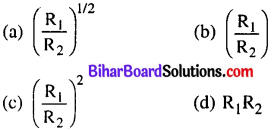(c)

Question 7.
Ampere is unit of
(a) Power
(b) Current
(c) Energy
(d) Potential
(b) Current

Question 8.
The S.I. unit of magnetic flux is
(a) Tesla
(b) Henry
(c) Weber
(d) Joule-sec
(c) WeberQuestion 9.
The working principle of Dynamo is based on
(a) Heating effect of current
(b) Electromagnetic induction
(c) On induced magnetism
(d) On induced current
(b) Electromagnetic induction

Question 10.
The hot wire Ammeter measure value of Alternating current
(a) Peak value
(b) Average value
(c) rms value
(d) None of these
(b) Average value

Question 11.
Which substance is suitable to made the core of transformer ?
(a) soft iron
(b) copper
(c) stainless steel
(d) Alnico
(a) soft ironQuestion 12.
The value of current decreases from 5A to OA in 0.1 sec. If induced emf is 200 V then value of self inductance is
(a) 4H
(b) 3H
(c) 4m H
(d) 3mH
(a) 4H

Question 13.
The change in magnetic field with time at a point produces
(a) Gravitational field
(b) Magnetic field
(c) Electric field
(d) None of these
(c) Electric field

Question 14.
Which have maximum penetrating power ?
(a) X-ray
(b) Cathode ray
(c) cc-fay
(d) y-ray
(d) y-rayQuestion 15.
A spherical mirror is immersed in water. Its focal length
(a) Increases
(b) Decreases
(c) Remains constant
(d) None of these
(c) Remains constant

Question 16.
A light rays goes from glass $$\left(\mu=\frac{5}{3}\right)$$ to water $$\left(\mu=\frac{4}{3}\right)$$ Its critical angle will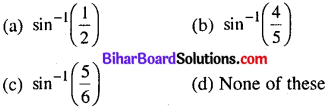(b)

Question 17.
The optical fibre works on the principle of
(a) scattering
(b) retraction
(c) dispersion
(d) total internal reflection
(d) total internal reflectionQuestion 18.
The unit of power of lens is
(a) Lambda
(b) Candela
(c) Dioptre
(d) Watt
(c) Dioptre

Question 19.
The image formed by simple microscope is
(a) Virtual and erect
(b) Inverted and virtual
(c) Real and erect
(d) Real and inverted
(a) Virtual and erect

Question 20.
Light waves are
(a) Transverse wave
(b) Longitudinal wave
(c) Progressive wave
(d) None of these
(a) Transverse waveQuestion 21.
The bending of light from obstacle is called
(a) Dispersion
(b) Diffraction
(c) Refraction
(d) Interference
(b) Diffraction

Question 22.
The momentum of photon which have 1 mega electron volt energy is
(a) 10-22 kg m/s
(b) 10-26 kg m/s
(d) 7 x 10-24 kg m/s
(c) 5 x 10-22 kg m/s
(c) 5 x 10-22 kg m/s

Question 23.
The energy of photon of wave length λ is
(a) he λ
$$\text { (b) } \frac{h c}{\lambda}$$
$$\text { (c) } \frac{h \lambda}{c}$$
$$\text { (d) } \frac{\lambda}{h c}$$
$$\text { (b) } \frac{h c}{\lambda}$$Question 24.
When a hydrogen atom is excited from its ground state to fourth orbit the maximum number of spectral line that can be emitted as
(a) 6
(b) 4
(c) 3
(d) 1
(a) 6

Question 25.
The unit of Rydberg’s constant is
(a) m-1
(b) m
(c) s-1
(d) s
(a) m-1

Question 26.
Which is chargeless particle ?
(a) a-particle
(b) P-particle
(c) proton
(d) photon
(d) photonQuestion 27.
α-rays are
(a) Electromagnetic wave
(b) Negative charged particle
(c) Positive charged particle
(d) Chargeless particle
(c) Positive charged particle

Question 28.
The mixing of impurity in semi conductor is called
(a) Doping
(b) Hybridization
(c) Sublimation
(d) None of these
(a) Doping

Question 29.
The current carriers are in N-type semi condutor is
(a) proton
(b) hole
(c) a-particle
(d) electron
(d) electronQuestion 30.
The resistance of semi conductor after increasing temperature
(a) increases
(b) decreases
(c) remains constant
(d) None of these
(b) decreases

Question 31.
p-n junction diodes are used as
(a) An amplifier
(b) Oscillator
(c) Modulator
(d) Rectifier
(d) Rectifier

Question 32.
P-type and N-type semi conductor are
(a) Electrically neutral
(b) Electrically positive
(c) Electrically negative
(d) None of these
(a) Electrically neutralQuestion 33.
The maximum distance upto which T.V. signal can be received from Antenna of height h is
$$(a) \sqrt{2 h} \mathrm{R} (b) h \sqrt{2 R}$$
$$\text { (c) } \mathrm{R} \sqrt{2 h}$$
(d) None of these
$$(a) \sqrt{2 h} \mathrm{R}$$

Question 34.
The polarisation of ground wave is
(a) in any direction
(b) at angle 60°
(c) perpendicular
(d) parallel
(c) perpendicular

Question 35.
The sun obtained radiant energy with
(a) Nuclear fission
(b) Nuclear disintegration
(c) Chemical reaction
(d) Nuclear fusion
(d) Nuclear fusionNon-Objective Type Questions

Question No. 1 to 18 are short answers type question. Each question carries 2 marks. Answer any ten (10) question.

Question 1.
Define dielectric constant and dielectric strength.
Dielectric constant:
The ratio of strength of applied electric field to the strength of reduced value of electric field on placing the dielectric b/w the plates of capacitor is called dielectric constant of dielectric medium. It is.also known as relative permitivity and denoted by K the value nx K is always greater than 1.

Dielectric Strength :
It is defined as the maximum value of electric field that can be applied to the dielectric without its electric breakdown.

S.I. unit of dielectric strength is Vm-1.Question 2.
Establish the relation between electric field (E) and potential (V)
$$\mathrm{E}=-\frac{d v}{d r}$$ Here, Negative sign indicates that when distance increases potential decreases.

The term $$\frac{d v}{d r}$$ is called potential gradient.

Question 3.
Write two uses of potentiometer.

• The electro motive force (emf) of two cells are compared by potentiometer,
• The internal resistance of cell is determined by potentiometer.

Question 4.
What is Seeback effect ?
The phenomenon of producing thermoelectric emf (or thermoelectric current in thermo couple) by keeping its two junctions at different temperature is called Seeback effect. The current produced in this way is called thermoelectric current and emf produced in this may be called thermoelectric emf.

Question 5.
Distinguish between electromagnet and permanent magnet.
Electro-magnet:

• Magnetic strength can be change
• The material of electro-magnet has high retentivity.
• The material of electro-magnet has low coericivity
• It has permeability.Permanent magnet :

• Magnetic strength is fixed,
• It has low retentivity.
• It has low coericivity
• It has permeability

Question 6.
What is Eddy currents ?
When magnetic flux linked with metallic conductor changes, induced current are set up in the conductor in the form of closed loops. These current looks like ‘eddies’ or ‘whirl pools’ and likewise are known as eddy currents.

Thus, eddy currents are the currents induced in a conductor, when placed in changing magnetic field.

Question 7.
What is Transformer ? Define transformation ratio.
Transformer:
It is a device which is well to convert low voltage at high current in high a.c. voltage at low current and vice-versa. It is based on the principle of mutual induction.

Transformation Ratio :
The ratio of number of turns of wire in secondary coil to the number of turns of wire in primary coil, is called transformation ratio.$$\frac{N s}{N p}=K$$ K is called Transformation Ratio

Question 8.
The radius of curvature of double convex lens are 10 cm and 15 cm. If focal length of limits is 12 cm, then find the refractive index of the material of the prism.
Given R1 = 10 cm, R2 = – 15 cm, f = 12 cm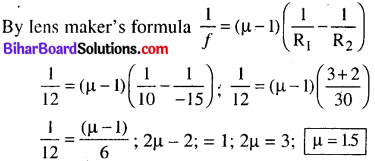Question 9.
What is critical angle ? And what are the conditions for total internal reflection ?
Critical Angle :
The angle in incidence corresponding to which angle of refraction becomes 90°, is called critical angle. It is denoted by ic

Conditions for total Internal Reflection :

• The light should travel from denser medium to rarer medium.
• The angle of incidence must be greater than critical angle for given pair of media..Question 10.
Write two properties of X-rays.

• They are not deflected by electric or magnetic field.
• They travel with the speed of light as electro¬magnetic wave.

Question 11.
State two properties of nuclear force.

• It is only attractive force,
• It is strong fundamental force in value/nature.

Question 12.
What is the de-Broglie associated with an electron, accelerated through a potential difference of 100 volt.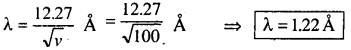Question 13.
What is photo electric effect ? Write photo electric equation.
The phenomenon of emission of electron from the metallic surface when suitable wave length of light is incident upon it, is called photoelectric effect. Photoelectric equation is
$$\frac{1}{2} m v^{2}=h v-h v_{0}$$Question 14.
Write two characteristics of LASER rays.

• It is highly directional with parallel beam.
• It is monochromatic and coherent.

Question 15.

• Light emitting diodes are easily manufactured.
• LEDs have 10W cost.
• It works at 10W voltage as compared to in- condescend bulb.
• No warm up time is taken by them.
• They can emit monochromatic light as well as bright light.

Question 16.
What will be the result if a student connects Voltmeter in series and Ammeter in parallel in circuit by mistake ?
By presence of Voltmeter in series with Resistance R in a circuit due to voltmeters high resistance, the current in the cirucuit I (= V/R) will decrease appreciably to I1 (= V/R) and as their I1 will further divide in Resistance R and ammeter, the currrent through ammeter I2 will be eveiv lesser than I1. Also the applied potential difference V will divide across resistance R and voltmeter, so the potential difference across voltmeter V2 will be lesser than V. Since I2 and V2 both are lesser than I and V respectively, none, of the instrument will be damaged.Question 17.
How do (a) the pole strength and (b) the magnetic moment of each part of bar magnet change, if is cut into two equal parts along its length.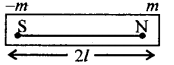Initially, Magnetic moment M = m.2l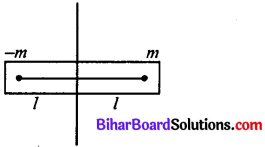In this position length becomes half then
$$M^{1}=m \cdot \frac{2 l}{2}=\frac{M}{2}$$

Polar strength remains same but magnetic moment becomes half of its initial value.Question 18.
Calculate induced emf in a moving conductor in an uniform magnetic field.
Let length of conductor PQ – 1
As x = 0, magnetic flux Φ = 0.
When PQ moves a small distance from x to x + dx, then magnetic flux linked = BdA = Bldx

The magnetic field is from x = 0 to x = b, so final magnetic flux = ΣBldx = Bl Σdx
= Blb (increasing)

Mean magnetic flux from x = 0 to x = b is 1/2 Bib.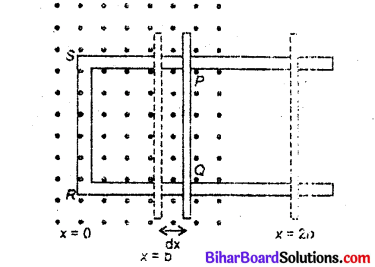The magnetic flux from x = b to x = 2b is zero. Induced emf,
$$\varepsilon=-\frac{d \phi}{d t}=-\frac{d}{d t}(B l d x)=-B l \frac{d x}{d t}=-B l v$$

where $$v=\frac{d x}{d t}$$ = velocity of arm PQ from x = 0 to x = b.
During return from x = lb to x = b the induced emf is zero; but now area is decreasing so magnetic flux is decreasing, and induced emf will be in opposite direction.Question No. 19 to 24 are long answers type question. Each question of this category carries 5 marks. Answer any three (3) question. (3 x 5 = 15)

Question 19.
Explain the principle on which Van de Graaff generator operates. Draw a labelled schematic sketch and write briefly its working.
Construction :
It consists of a large hollow metallic sphere S mounted on two insulating columns A and B and an endless belt of rubber or silk is made to run on two pulleys P1 and P2 by the means of an electric motor. C1 and C2 are two sharp metallic spikes in the form of combs. The lower comb C1 is connected to the positive terminal of a very high voltage source (HTS) (= 104 volts.) and the upper comb C2 is connected to the inner surface of metallic sphere S.

Working:
When comb C1 is given very high potential then it produces ions in its vicinity, due to action of sharp points. The positive ions, so produced, get sprayed on the belt due to repulsion between positive ions and comb C1. These positive ions are carried upward by the moving belt. The pointed end of C2 just touches the belt. The comb C2 collects positive charge from the belt which immediately moves to the outer surface of sphere S. As the belt goes on revolving, it continues to take (+) charge upward which is collected by comb C2 and transferred to outer surface of sphere S. Thus the outer surface of metallic sphere S gains positive charge continuously and its potential rises to a very high value.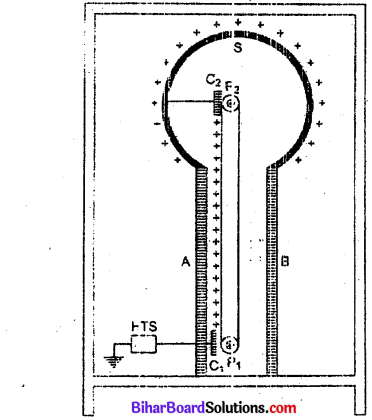When the potential of a metallic sphere gains very high value, the dielectric strength of surrounding air breaks down and its charge begins to leak, to the surrounding air. The maximum potential is reached when the rate of leakage of charge becomes equal to the rate of charge transferred to the sphere. Thus, the high voltages can he built up to breakdown field of the surrounding medium. To prevent leakage of charge from the sphere. the generator is completely enclosed in an earthed connected steel tank which is filled with air under high pressure.

Van de Graaff generator is used to accelerate stream of charged particles to very high velocities. Such a generator is installed at ¡IT Kanpur which accelerates charged particles upto 2 MeV energy.

Question 20.
(a) State the principle of working of a potentiometer.
(b) Draw a circuit diagram to compare the cmf of two primary cells. Write the formula used. How can the sensitivity of a potentiometer be increased?
Principle of Potentrometer:
Principle: It is based on the principle that when constant current passes from unifòrm cross sectional area of wire thus potential drop across any portion f wire is directly proportional to length of that portion of wire.

V ∝ l

Comparison of emPs òf two cells:
First of all the ends of potentiometer are connected to a battery B1, key K and Rheostate Rh such that the positive terminal of battery 1 is connected to end A of the wire. This completes the primary circuit.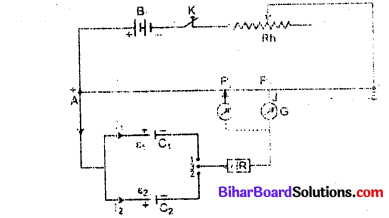Now the positive terminals of the cells C1 and C2 whose emfs are to be compared are connected to A and the negative terminals to the jockey J through a two way key and a galvanometer (fig). This is the secondary circuit.

Method :
(i) By closing key K, a potential difference is established and rheostat is so adjusted that when jockey J is made to touch at ends A and B of wire, the deflection in galvanometer is on both sides. Suppose in this position the potential gradient is k.

(ii) Now plug is inserted between the terminals 1 and 3 so that cell C j is included in the secondary circuit and jockey J is slided on the wire at Pj (say) to obtain the null point. The distance of P, from A is measured. Suppose this length is l1 i.e. AP1 = l1.

∴ The emf of cell C1, ε1 = kl1 …………….. (i)

(iii) Now plug is taken off between the terminals 1 and 3 and inserted in between the terminals 2 and 3 to bring cells C2 in the circuit. Jockey is slided on the wire and null deflection position P2 is noted. Suppose distance of P2 from A is I2 i.e., AP-) = l2
The emf of cell C2, ε2 = d2 ………………(ii)
Dividing (i) by (ii), we get
$$\frac{\varepsilon_{1}}{\varepsilon_{2}}=\frac{l_{1}}{l_{2}}$$ ………….(iii)

Thus emfs of cells may be compared. Out of these cells if one is standard cell, then the emf of other cell may be calculated.Question 21.
State KirchofTs law. Write its one application based on this law (Wheatstone bridge).
Kirchoff’s law : First law :
In the network of conductor the algebraic sum of current meeting at a point is always zero. It also causes junction rule.

Second law:
It states that in any closed part of an electrical circuit, the algebraic sum of the emf is equal to the algebraic sum of the products of the resistance and current flowing through them. It is also called loop rule. This law is based on the law of conservation of energy.

Wheatstone bridge :
It is one of the accurate arrangements for measuring an unknown resistance. It is based on the principle of Kirchoff s law.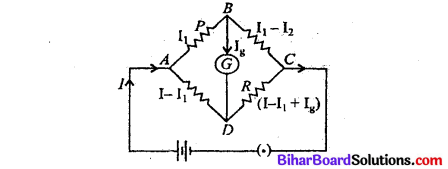Four resistance P, Q, R and S are connected to form a quadrilateral of galvanometer and a tapping key kl (called galvanometer) are connected between points B and D as shown in the figure.

Applying Kirchoff s second law, for closed part ABDA in the circuit I1 P + Ig. g – (I – I1) S = 0

where g is the resistance of the galvanometer
In the balanced state Ig = 0Applying Kirchoff s law in closed part BDCB
Ig. g + (I – I1 + Ig) R – (I1 – Ig) Q = 0
O + (I – I1) R – I1 Q = 0
(I – I1) R = I, Q
$$\frac{Q}{R}=\frac{I-I_{1}}{I_{1}}$$ ………………… (ii)From equation (i) and (ii)
$$\frac{P}{S}=\frac{Q}{R}\left[S=\frac{P}{Q} \cdot R\right]$$

Thus unknown resistance can be calculated.

Question 22.
Draw a ray diagram for formation of image of a point object by a thin double convex lens having radii of curvature Rj and R2. Hence, derive lens maker’s formula for a double convex lens. State the assumptions made and sign convention used.

Or, Using the relation for refraction at a single spherical refracting surface, derive the lens makers formula.
Lens Maker’s Formula :
Suppose L is a thin lens. The refractive index of the material of lens is n2 and it is placed in a medium of refractive index n1. The optical centre of lens is G and X. X is principal axis. The radii of curvature of the surfaces of the lens are R1 and R2 and their poles are P1 and P2. The thickness of lens is t, which is very small. O is a point object on the principal axis of the lens. The distance of O from pole P1 is u. The first refracting surface forms the image of O at I’ at a distance v’ from P1.

From the refraction formula at spherical surface,
$$\frac{n_{2}}{v^{\prime}}-\frac{n_{1}}{u}=\frac{n_{2}-n_{1}}{\mathrm{R}_{1}}$$ ………………………. (i)The image I’ acts as a virtual object for second surface and after refraction at second surface, the final image is formed at I. The distance of I from pole P2 of second surface is v. The distance of virtual object (T) from pole P2 is (v’ -t).For refraction at second surface, the ray is going from second medium (refractive index n1) to first medium (refractive index n2), therefore from refraction formula at spherical surface.

$$\frac{n_{1}}{v}-\frac{n_{2}}{\left(v^{\prime}-t\right)}=\frac{n_{1}-n_{2}}{\mathrm{R}_{2}}$$ ……………………. (ii)

For a thin lens t is negligible as compared to v’, therefore from (ii)where $$\mathrm{l} n_{2}=\frac{n_{2}}{n_{1}}$$ is refractive index of second medium (i.e. medium of lens) with respect to first medium.

If the object O is at infinity, the image will be formed at second focus i.e.,

if u = α, v = f2 = f, Therefore from equation (iv)This is the formula of refraction for a thin lens. This formula is called Lens-Maker’s formula.

If first medium is air and refractive index of material of lens be n, then 1n2 = n, therefore equation (v) may be written as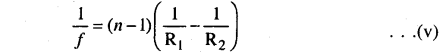Question 23.
(a) With the help of a diagram, explain the principles and working of a moving coil galvanometer.
(b) What is importance of a radial magnetic field and how is it produced?
(a) Moving coil galvanometer : A galvanometer is used to detect current in a circuit.

Construction :
It consists of a rectangular coil around on a non-conducting metallic frame and is suspended by phosphor bronze strip between the pole pieces (N and S) of a strong permanent magnet.A soft iron core in cylindrical form is placed between the coil.

One end of coil is attached to suspension wire which also serves as one terminal (T1) of galvanometer. The other end of coil is connected to a loosely coiled strip which serves as the other terminal (T2). The other end of the suspension is attached to a torsion head which can be rotated to set the coil in zero position. A mirror (M) is fixed on the phosphor bronze strip by means of which the deflection of the coil is measured by the lamp and scale arrangement. The levelling screws are also provided at the base of the instrument.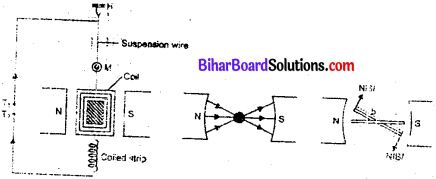The pole pieces of the permanent magnet are cylindrical so that the magnetic field is radial at any position of the coil.

Principle and working :
When current (I) is passed in the coil, torque t acts on the coil, given by τ = NIABsinθ where θ is the angle between the normal to plane of coil and the magnetic field of strength B, N is the number of turns in a coil.

When the magnetic field is radial, as in the case of cylindrical pole pieces and soft iron core, then in every position of coil the plane of the coil, is parallel to the magnetic field lines, so that 0 = 90° and sin 90° = Deflecting torque, τ = NIAB

If C is the torsional rigidity of the wire and 0 is the twist of suspension strip, then restoring torque = CθFor equilibrium, deflecting torque = restoring torque
i.e., NIAB = Cθ
∴ $$\quad \theta=\frac{N A B}{C} I$$ ………………. (i)
i.e, θ ∝ I

deflection of coil is directly proportional to current flowing in the coil and hence we can construct a linear scale.

(b) (i) Importance (or function) of uniform radial magnetic field : Torque as current carrying coil in a magnetic field is θ = NIAB sin θ
In radial magnetic field sin θ = 1, so torque is τ = NIAB.

This makes the deflection (θ) proportional to current. In other words, the radial magnetic field makes the scale linear.

(ii) Function of soft iron core : To produce radial magnetic field pole pieces of permanent magnet are made cylindrical and a soft iron core is placed between them. The soft iron core helps in making the field radial and reduce energy losses produced due to eddy currents.

Question 24.
Using the postulates of Bohr’s model of hydrogen atom, obtain an expression for the frequency of radiation emitted when atoms make a transition from the higher energy state with quantum number ni. to the lower energy state with quantum number nf (nf < ni)
Suppose m be the mass of an electron and e its speed in nth orbit of radius r. The centripetal force for revolution is produced by electrostatic attraction between electron and nucleus.Again from Bohr’s postulate for quantization of angular momentum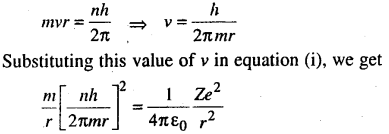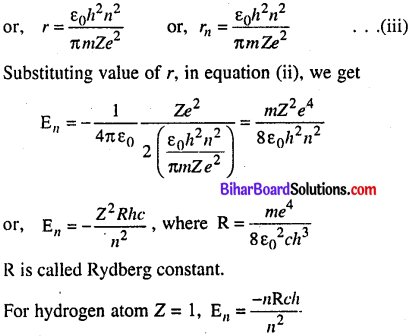If ni and nf are the quantum numbers of initial and final states and Ei & Ef are energies of electron in H-atom in initial and final state, we have
$$\mathrm{E}_{i}=\frac{-\mathrm{R} h c}{n_{i}^{2}} \text { and } \mathrm{E}_{f}=\frac{-\mathrm{R} h c}{n_{f}^{2}}$$

If v is the frequency of emitted radiation, we get $$v=\frac{\mathrm{E}_{i}-\mathrm{E}}{h}$$
$$v=\frac{-\mathrm{R} c}{n_{i}^{2}}-\left(\frac{-R c}{n_{f}^{2}}\right) ; \quad v=\mathrm{R} c\left[\frac{1}{n_{f}}-\frac{1}{n_{i}^{2}}\right]$$

error: Content is protected !!